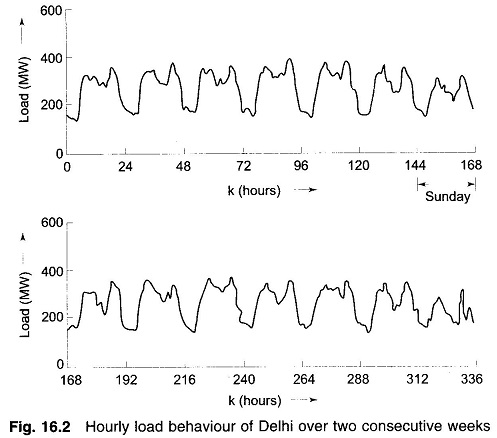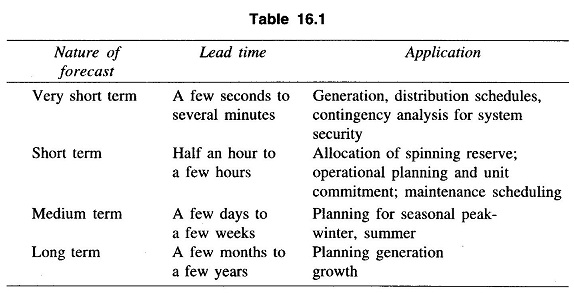## Long Term Load Forecasting in Power System

Long Term Load Forecasting in Power System: Long Term Load Forecasting – If the load forecasts are required for planning purposes, it is necessary to select the lead time to lie in the range of a few months to a few years. In such cases, the load demand should be decomposed in a manner that …

## Kalman Filtering Approach

Kalman Filtering Approach: The time series approach has been widely employed in dealing with the load forecasting problem in view of the relative simplicity of the model forms. However, this method tends to ignore the statistical information about the load data which may often be available and may lead to improved load forecasts if utilized …

## Time Series Forecasting Methods

Time Series Forecasting Methods: If yd(k) is subtracted from the sequence y(k), the result would be a sequence of data for the stochastic part of the load. We have to identify the model for ys(k) and then use it to make the prediction ys(k + j). A convenient method for this is based on the …

## Estimation Techniques of Periodic ComponentsEstimation Techniques of Periodic Components: Estimation Techniques of Periodic Components – The deterministic part of the load may contain some periodic components in addition to the average and the polynomial terms. Consider for example the curve shown in Fig. 16.2 which gives the variation of the active power supplied by a power utility over a …

## Estimation of Trend Analysis in Load Forecasting

Estimation of Trend Analysis in Load Forecasting: Estimation of Trend Analysis in Load Forecasting – The simplest possible form of the deterministic part of y(k) is given by where y̅d represents the average or the mean value of yd(k), bk represents the `trend’ term that grows linearly with k and e(k) represents the error of modelling …

## Types of Forecasting Methods

Types of Forecasting Methods: Types of Forecasting Methods may be divided into three broad classes. Techniques may be based on extrapolation or on correlation or on a combination of both. Techniques may be further classified as either deterministic, probabilistic or stochastic. Extrapolation Techniques: Extrapolation techniques involve fitting trend curves to basic historical data adjusted to …

## What is Load Forecasting in Power System?What is Load Forecasting in Power System?: Load Forecasting in Power System plays an important role in power system planning, operation and control. Forecasting means estimating active load at various load buses ahead of actual load occurrence Planning and operational applications of load forecasting requires a certain ‘lead time’ also called forecasting intervals. Nature of …

Scroll to Top